﻿ Stable Laws and the Number of Ordinary

### Stable Laws and the Number of Ordinary

P.M. MazurkinOPEN ACCESSPEER-REVIEWED

## Stable Laws and the Number of Ordinary

Doctor of Engineering Science, Academician of RANS, member of EANS, Volga Region State Technological University, Russia

### Abstract

Power total number of primes from the discharge of the decimal system is identified by the law of exponential growth with 14 fundamental physical constants. Model obtained on the parameters of the physical constants, proved less of the error and it gives more accurate predictions of the relative power of the set of prime numbers. The maximum absolute error of power (the number of primes), the traditional number is three times higher than suggested by us complete a number of prime numbers. Therefore, the traditional number 2, 3, 5, 7,. .. is only a special case. The transformation In10=2,30285… it was a rough rounded, leading to false identification of physico-mathematical regularities of different series of prime numbers. Model derived from physical constants, proved more accurate than the relative accuracy, and it gives more accurate predictions of the relative power of the set of prime numbers with increasing discharge the decimal number system.

### At a glance: Figures

123
Prev Next

• Mazurkin, P.M.. "Stable Laws and the Number of Ordinary." Applied Mathematics and Physics 2.2 (2014): 27-32.
• Mazurkin, P. (2014). Stable Laws and the Number of Ordinary. Applied Mathematics and Physics, 2(2), 27-32.
• Mazurkin, P.M.. "Stable Laws and the Number of Ordinary." Applied Mathematics and Physics 2, no. 2 (2014): 27-32.

 Import into BibTeX Import into EndNote Import into RefMan Import into RefWorks

### 1. Introduction

Prime number - is a natural numberthat has two positive divisors: one and itself.

There are several variants of distribution or a series of prime numbers (SPN):

1) finite number of critical primes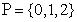;

2) non-critical prime numbers;

3) the traditional  number of primes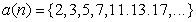with order (serial number), which was considered by many scientists and by Riemann;

4) part series of prime numbers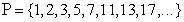;

5) the total number of prime numbersthat are equivalent row.

The literature focuses on SPN3, and we did not find sufficient publications on the analysis of SPN4 and other ranks have been proposed by us. In this reader a series of five articles examined SPN1, SPN2 SPN5 and compared with evidence SPN3.

### 2. Methodology

The main type of natural numbers are in (0;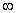). If we miss this kind of integers due to rejection of negative numbers. For convenience, we will use mathematical analysis, recording the results of calculations in the form of rational numbers just to prove the Riemann hypothesis. Quantitative analysis is a leap to mind the actual (real) numbers, according to the scheme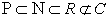without taking into account the complex numbers, but with irrational numbers such as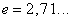and(18 characters after the decimal point in the software environment CurveExpert) and other fundamental constants.

In the analysis of stable laws have been applied  to the distribution of prime numbers.

### 3. Biotechnical Law and Its Fragments

Under the scheme "from the simple to the complex structure" in Table 1 are all stable laws are used to construct formulas biotech laws. Generalizing formula is biotech law . Most often, the sum of two biotech laws constitutes a deterministic allocation model.

Formula, together with a finite set SPN runs in a software environment CurveExpert for parameter identification of a stable law and wave patterns. Search for the model parameters is called the structural-parametric identification. For the processes of behavior of living and/or inert substances (according to V.I. Vernadsky) parameters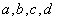biotech law and its fragments may approach to the fundamental physical constants, and it has been shown in the distribution of chemical elements .

### 4. Power Series of Prime Numbers

According to  SPN3 and our calculations on SPN5 in Table 2 shows the cardinal numbers and their relationships SPN5/SPN3.

#### Table 2. The relative cardinal number the increase in the capacity (quantity) of prime numbers

In the first digit decimal numbers the difference between a full and traditional rows of simple number is equal to 150 %. The relative cardinal number is the maximum 100,31 at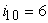and minimum 66.67 at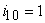. What SPN better? In advance, we say that SPN5.

With the increase in decimal place of natural numbers the increase in the relative cardinal number of the set of prime numbers with a capacity of more than 455 million occurs (Figure 1) by a deterministic model of the law of exponential growth.(1)
Figure 1. The schedule of the law of exponential growth (1) the relative power and remains after it: S - dispersion; r- correlation coefficient

On the balances was obtained (Figure 2) of the wavelet function (described in the second article)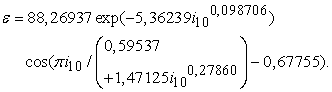(2)

The law of exponential death before the cosine function shows half of the amplitude of the oscillatory perturbations of power SPN3. Because of the high value of the remainder for, we have that zero discharge is theoretically possible number of prime numbers must be 88.

Combining formulas (1) and (2) gives the binomial model with the wave function (Figure 3) of the form(3)

Top of the wave has moved up to 957 prime numbers with zero discharge of the decimal system. In addition, under the function of the cosine of half-cycle fluctuations has changed: the beginning shifted to the first digit of the negative numbers. Half-life increases sharply, and the intensity parameter of death -0.33681 shows anomalous behavior of the model (3).

From the graph in Figure 3 we can see that the shape of the curve repeats part of the curve of the Riemann zeta-function, located in the positive area of complex numbers.

### 6. Full Range

This SPN5 received a deterministic pattern (Figure 4) the type of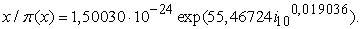(4)

Residues have a relatively smooth swing and determined (Figure 5) by the formula: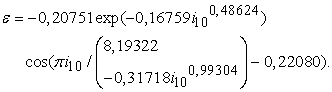(5)

In a number of natural numbers 0, 1, 2, 3, 4, 5, 6, 7, 8, 9, there are six prime numbers, three of which (0, 1, 2) critical (negative sign before the formula vibration), and three numbers (3, 5, 7) - a non-critical. At zero there is no prime numbers, so the law of exponential growth begins with a small actual (real) numbers, and -0.20751.

In formula (5), half of the amplitude of the perturbations of the power SPN5 has the numerical value of all 0,20751. The initial half-life 8,19322 damped oscillations approaching 8.

We express the frequency response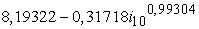of the oscillatory disturbance is half-wave decline, i.e. with increasing discharge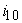is the growth rate fluctuations on the scale of natural numbers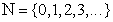, and it - the effect of the tremor.

The general equation (Figure 6) is characterized by binomial formula(6)

Remains of the formula (6) are so small that, as seen in the upper right corner in Figure 6, the variance of the residual is zero and the correlation coefficient is unity.

Comparison of the remains of the formulas (3) and (6) shown in Figure 7.

The maximum absolute error of power (the number of primes), the traditional number is three times higher compared to the total number of primes.

Then it turns out that the traditional number is only a special case.

### 7. Do not Change the Scale of Reference of Natural Primes

This recommendation for the future in the study of prime numbers comes from the fact that, from Riemann used the natural logarithm and are looking for an empirical formula . To quote from an article by Don Zagier:

"Apparently (see Table 2.), that the ratio of x to π(x) the transition from a given degree of ten to follow all the time increases to about 2.3. Mathematics is recognizable among the 2.3 log 10 (of course, to base e). The result suggested that the~, where the sign ~ means that the ratio of their expressions are connected with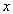tends to 1. This asymptotic equation, first proved in 1896, is now the law of distribution of prime numbers. Gauss, the greatest of mathematicians, discovered this law in the age of fifteen, studying tables of primes contained in the gift to him a year before the table of logarithms".

We were not too lazy to check the statement "the ratio of x to π (x) in the transition from the present level of ten to follow all the time increases by about 2.3" and the results of the calculations resulted in Table 3. Here, the number 2.30 in SPN3 not (if there is, the approximation error to 2.30 at 100(2.5 - 2.3) / 2.3 = 8.70%, which is very much), but there is an aspiration to 1. At the same time the full range of gives at the beginning of the interval of digits in a larger multiplicity 2,22 (error of 3.47 %).

Equal to the power of two sets SPN3 and SPN5 can be considered, starting with the digitsin decimal notation.

With the growth ofa true statement is the convergence to 1. For this purpose we identify the law of death (in a general form of Table 1) according to the statistical data of Table 3.

#### Table 3. The multiplicity of cardinal number

For the full range of the obtained formula(7)

Equation (7) shows that the ratio of cardinal numbers will not come near to the unit and can only reach the values of the 1,0998.

From the article  reads: «After more than a careful and complete calculation, Legendre in 1808 found that particularly good approximation is obtained if we subtract from ln x is not 1, but 1.08366, i.e.~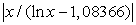». In the formula (7) the constant 1.09980 is little different.

Thus, number of prime numbers, the power has been studied in a number system with base= 2.718281828. .... It is known that this system has the greatest density of information recording and refers to the nonintegral positional systems. But non-integers do not belong to the natural numbers, let alone to a series of prime numbers.

Thus, the transformationit was a rough rounded, leading to false identification of physico-mathematical regularities of different series of prime numbers.

With "easy" hands Gauss in mathematics, vigorously developed the theory of approximation, which made it possible to linearize the scale of the abscissa and ordinate in terms of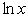and. Thus is the fundamental transformation of the statistical data presented at the beginning of the decimal system, in logarithmic. As a result, the closed form of design patterns that are not only difficult to understand, but they have lost and the visibility of graphics and even more so - the physical representation. Therefore, we continue to recommend in its publications to readers an open system of mathematical constructs according to the laws of Table 1.

### 8. Fundamental Constants

Formulas from Table 1 gives the identification of fundamental physical constants to the parameters. Processes themselves are unknown.

Carefully consider the formula (4), and compare the values of parameters of the mathematical model with the fundamental constants. Recall that Don Zagier  analyzed (see Table 2) a very large number of natural numbers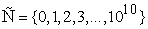with a finite numberof prime numbers and gave them a set of up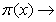455 052 512.

We put forward a hypothesis (Table 4): with an increase in the relative power of the total number of prime numbers, the parameters of the model (4) will tend to the fundamental constant .

To a first approximation we replace the law (4) to the physical equivalent to the formula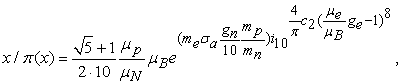(8)

legend of the model parameters (8) are given in Table 4 (10 - radix).

### 9. The Law with the Fundamental Constants

After substituting the fundamental physical constants in Table 4 we write the model (8) as a law of exponential growth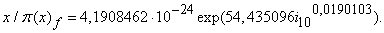(9)

Next check the adequacy of the models (4) and (9). Known formulas allowing to calculate the number of primes faster. In this way, it was calculated that up to 1023 is 1 925 320 391 606 803 968 923 primes.

Then we get to the data  the new value51,9394 (Table 5).

Model (9), obtained from the physical constants in Table 4, was even more precise on the relative error, and it gives more accurate predictions of the relative power of the set of prime numbers.

The error for the arrayis equal to only 0,08 %.

By the remnants of (9) is obtained (Figure 8) the equations of the perturbation.

#### Table 5. The adequacy of the law of exponential growth

Figure 8. Diagrams of the perturbation capacity of prime numbers depending on the order of the decimal system

Biotechnical law as a supplement to (9) shows that after the discharge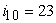in the relative power is going on a decline. Damped oscillation shows that with increasing power of primes wavetends to zero. Whenthe perturbation is almost excluded.

### 10. Conclusions

Power total number of primes from the discharge of the decimal system is identified by the law of exponential growth to the fundamental physical constants. With the growth of the power of the prime numbers increases the adequacy of equation (8) with the physical constants, which can lead in the future to the general equation four interactions.

### References

  Don Zagier. The first 50 million prime numbers. URL: http://www.ega-math.narod.ru/Liv/Zagier.htm.In article  Number. URL: http://ru.wikipedia.org/wiki/%D0%A7%D0%B8%D1%81%D0%BB%D0%BE.In article  Mazurkin P.M. Biotechnical principle and sustainable laws of distribution // Successes of modern natural sciences. 2009. № 9, 93-97.In article  Mazurkin PM The statistical model of the periodic system of chemical elements D.I. Mendeleev. Yoshkar-Ola: MarSTU, 2006. 152.In article  Fundamental physical constants. URL; http://www.akin.ru/spravka/s_fund.htm.In article